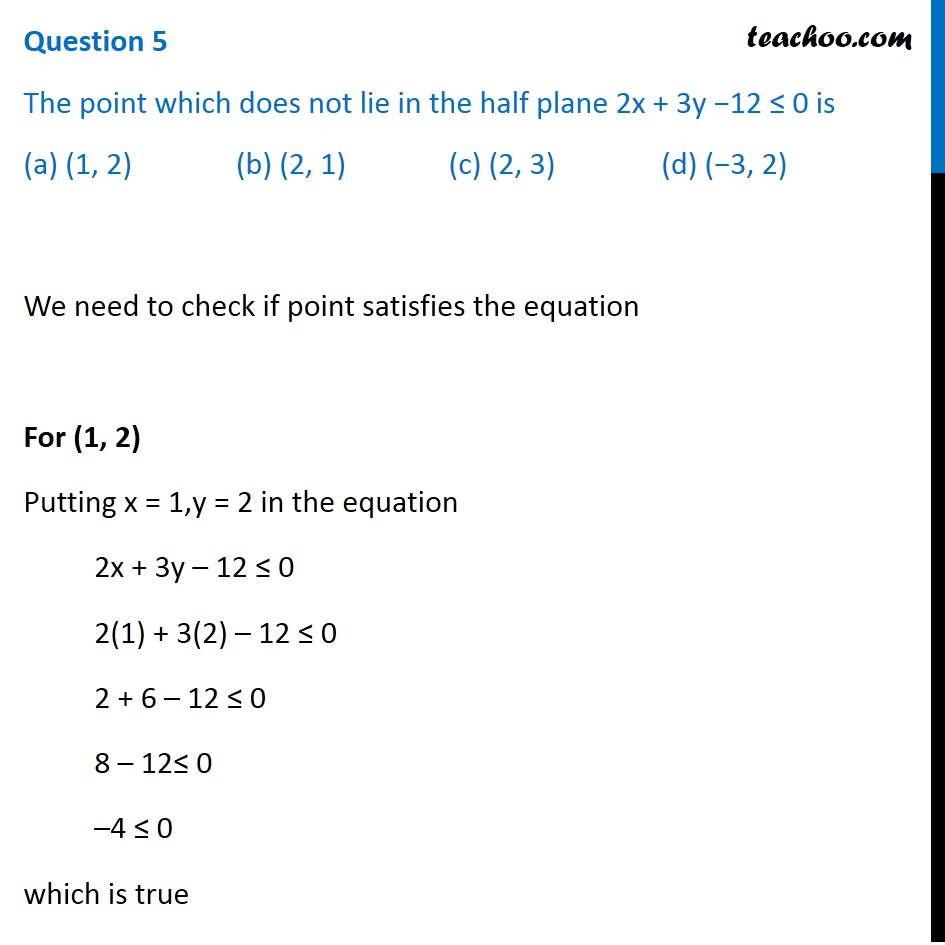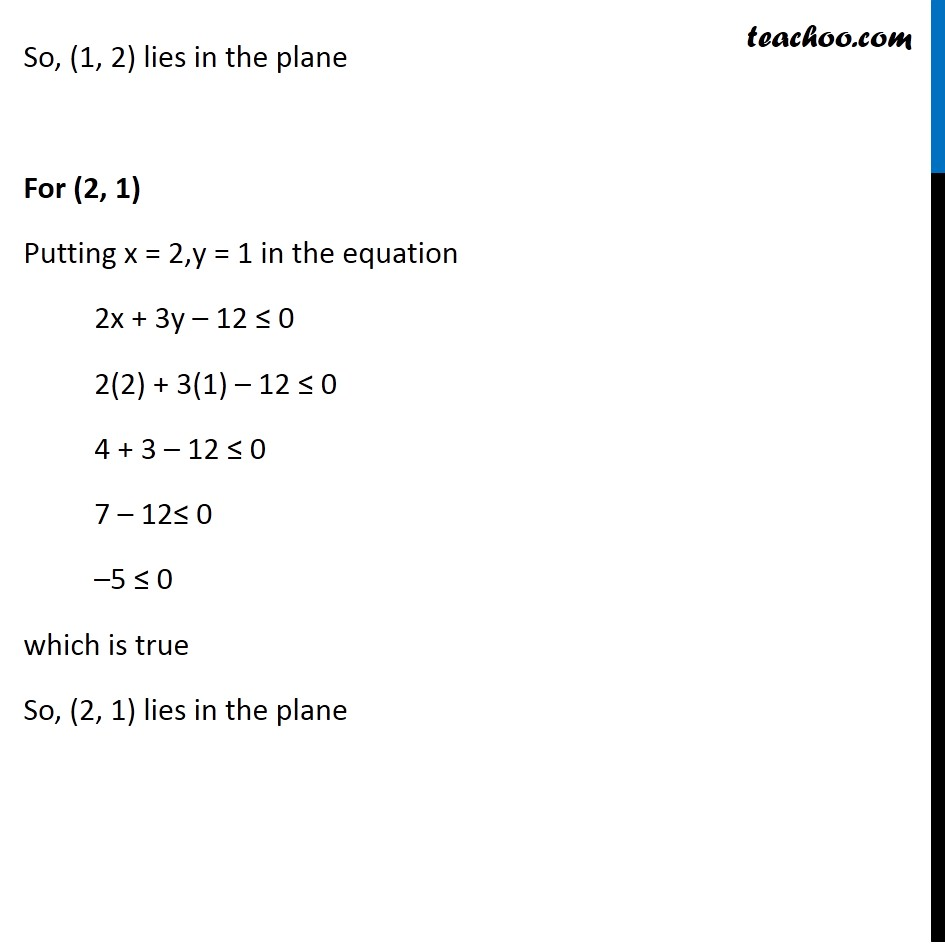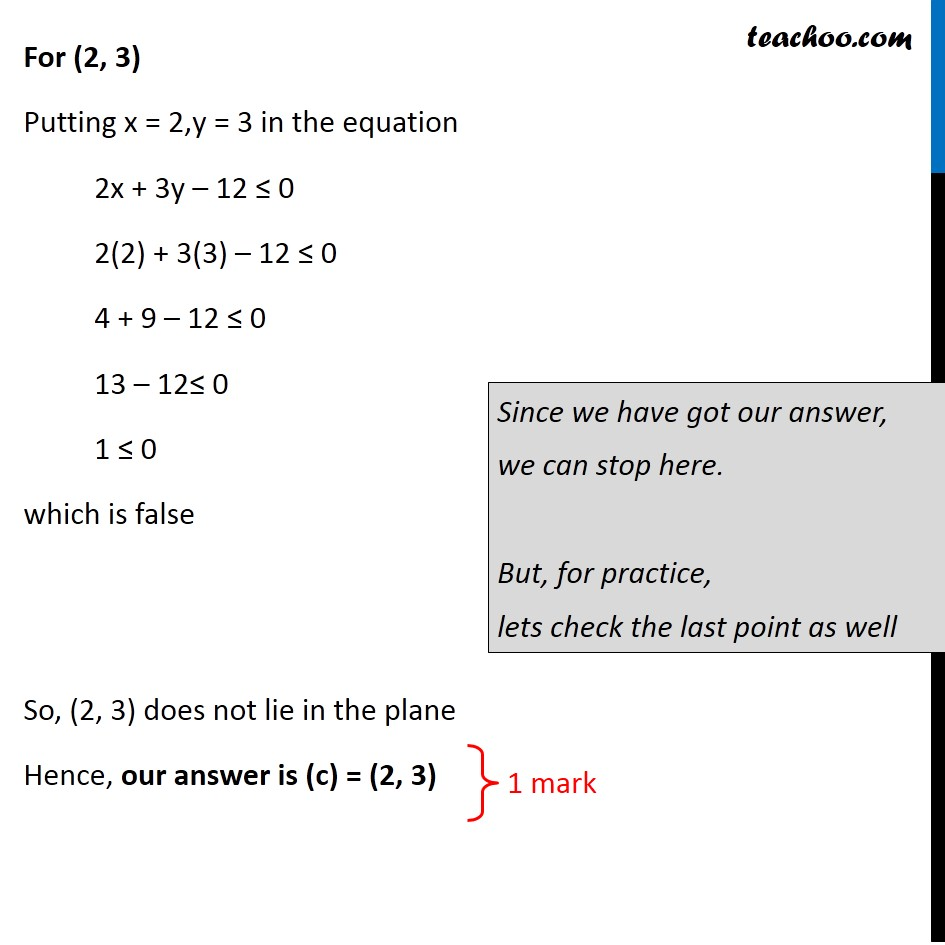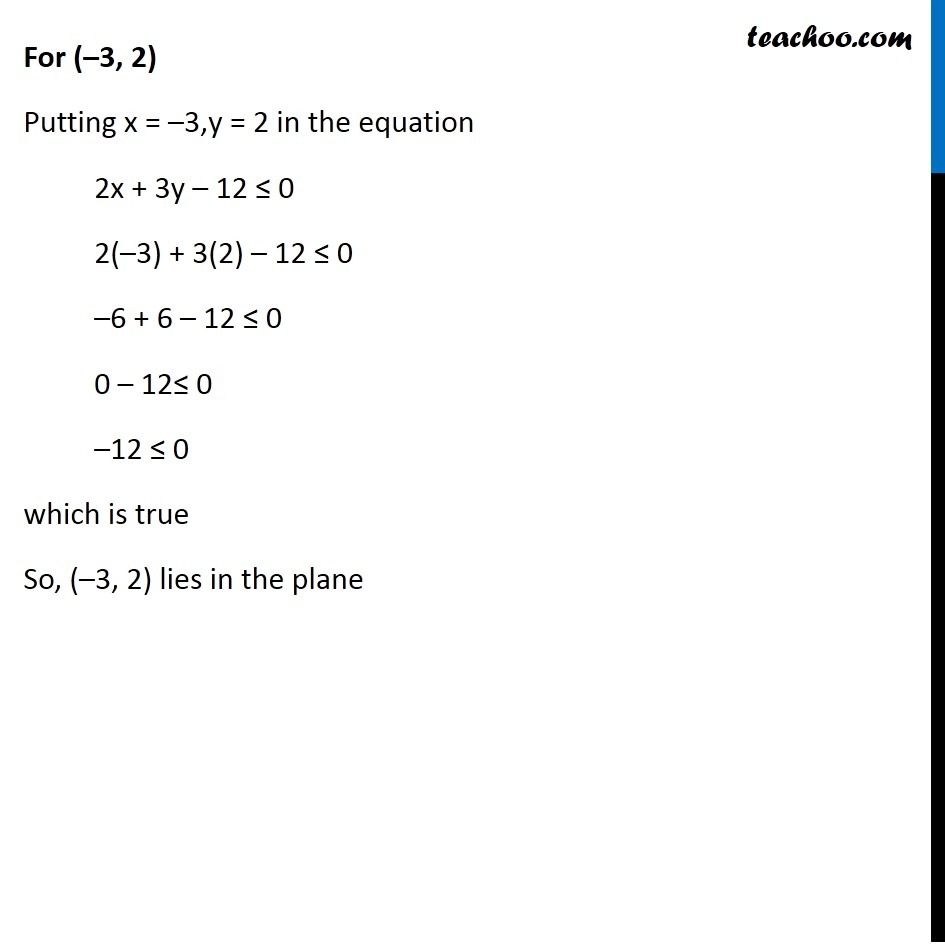CBSE Class 12 Sample Paper for 2020 Boards

Class 12
Solutions of Sample Papers and Past Year Papers - for Class 12 Boards

## (a) (1, 2)                  (b) (2, 1)                 (c) (2, 3)             (d) (−3, 2)Learn in your speed, with individual attention - Teachoo Maths 1-on-1 Class

### Transcript

Question 5 The point which does not lie in the half plane 2x + 3y −12 ≤ 0 is (a) (1, 2) (b) (2, 1) (c) (2, 3) (d) (−3, 2) We need to check if point satisfies the equation For (1, 2) Putting x = 1,y = 2 in the equation 2x + 3y – 12 ≤ 0 2(1) + 3(2) – 12 ≤ 0 2 + 6 – 12 ≤ 0 8 – 12≤ 0 –4 ≤ 0 which is true So, (1, 2) lies in the plane For (2, 1) Putting x = 2,y = 1 in the equation 2x + 3y – 12 ≤ 0 2(2) + 3(1) – 12 ≤ 0 4 + 3 – 12 ≤ 0 7 – 12≤ 0 –5 ≤ 0 which is true So, (2, 1) lies in the plane For (2, 3) Putting x = 2,y = 3 in the equation 2x + 3y – 12 ≤ 0 2(2) + 3(3) – 12 ≤ 0 4 + 9 – 12 ≤ 0 13 – 12≤ 0 1 ≤ 0 which is false So, (2, 3) does not lie in the plane Hence, our answer is (c) = (2, 3) Since we have got our answer, we can stop here. But, for practice, lets check the last point as well For (–3, 2) Putting x = –3,y = 2 in the equation 2x + 3y – 12 ≤ 0 2(–3) + 3(2) – 12 ≤ 0 –6 + 6 – 12 ≤ 0 0 – 12≤ 0 –12 ≤ 0 which is true So, (–3, 2) lies in the plane